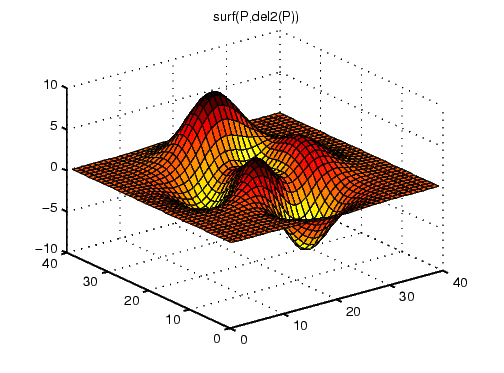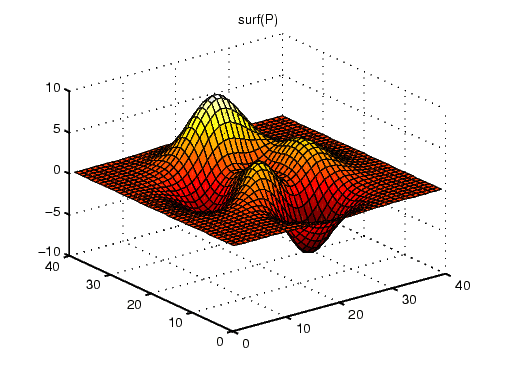3-D VisualizationExample -- Mapping Surface Curvature to Color

The Laplacian of a surface plot is related to its curvature; it is positive for functions shaped like `i^2 + j^2` and negative for functions shaped like `-(i^2 + j^2)`. The function `del2` computes the discrete Laplacian of any matrix. For example, use `del2` to determine the color for the data returned by `peaks`.

• ```P = `peaks`(40);
C = del2(P);
surf(P,C)
colormap hot
```

Creating a color array by applying the Laplacian to the data is useful because it causes regions with similar curvature to be drawn in the same color.Compare this surface coloring with that produced by the statements

• ```surf(P)
colormap hot
```

which use the same colormap, but map regions with similar z value (height above the x-y plane) to the same color.Indexed Color Surfaces -- Direct and Scaled Colormapping Altering Colormaps© 1994-2005 The MathWorks, Inc.